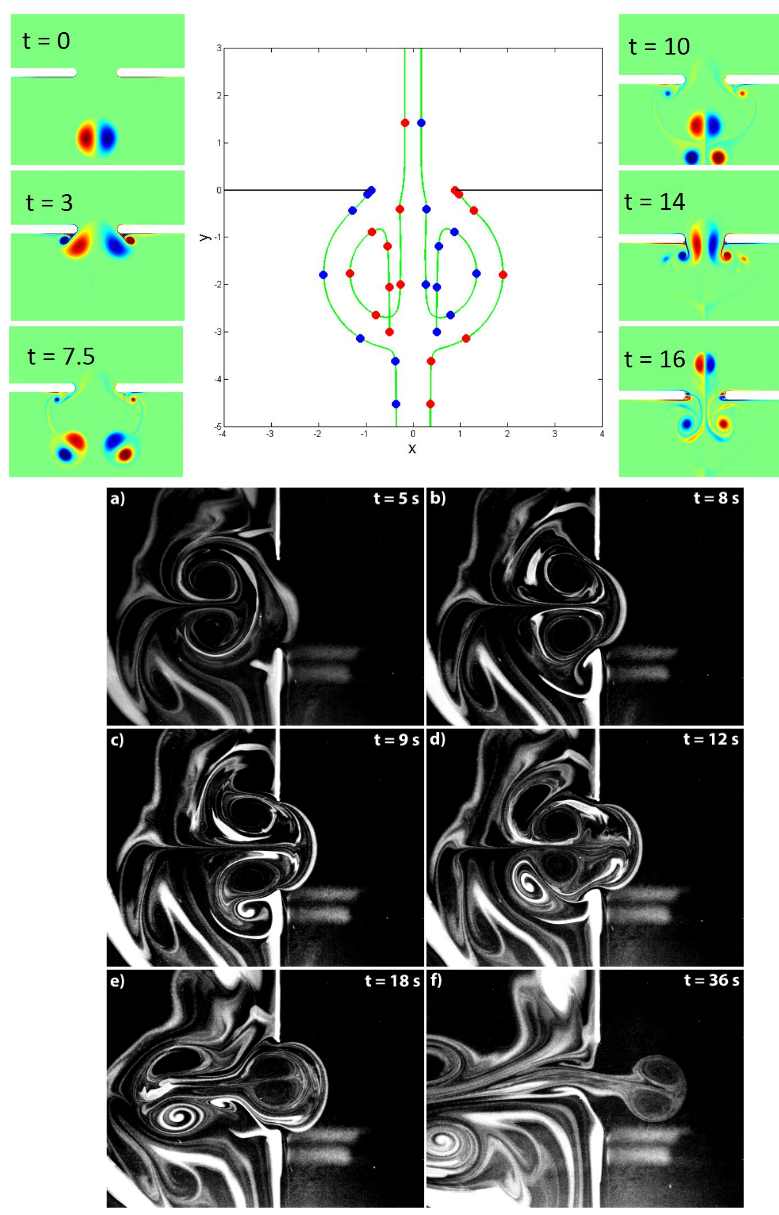# A dipolar vortex at the approach of an opening.

On this page, the dynamical behaviour of a dipolar vortex that encounters an opening between two peninsulalas is studied. Inspiration comes from C.H. Wong’s (2015) and my (van Hooft, 2015) master thesis projects.

The boundary is embedded into the (Navier-Stokes) flow domain. Furthermore, we will review our results.

#include "embed.h"
#include "navier-stokes/centered.h"
#include "view.h"

The location of the peninsulas ends and half widths are set. Furthermore, the dipole’s starting position is defined.

double xpl = 1.2, ypl = 0.12, wpl = 0.2;
double xd = 0.0, yd = -5;

Adaptive mesh-element-size selection is carried out via the wavelet-estimated discretization error. The balance between accuracy and speed performance may be tuned via the refinement criterion for the velocity components (ue) and the maximum level of the tree-grid refinement (maxlevel).

double ue = 0.025;  // = U/40
int maxlevel = 12;  // Max 4096 x 4096 cells
return adapt_wavelet((scalar*){cm, u}, (double[]){0.01, ue, ue},
maxlevel).nf;
}

With the dipole’s radius (R), propagation velocity (U) and the fluid’s vicosity (nu), a reynolds number can be readily defined as Re = \frac{UR}{\nu}. Its value is defined bleow and the domain size is set to be 30R \times 30R.

face vector muc[];
double Re = 3000.;

int main(){
L0 = 30;
X0 = Y0 = -L0/2;
N = 256;
mu = muc;
run();
}

event properties (i++){
foreach_face()
muc.x[] = fs.x[]/Re;
boundary ((scalar*){muc});
}

## Initialization

The embedded boundary is defined and the flow field is initialized with a Lamb-chaplygin dipolar vortex. The grid is initialized consistent with the aformentioned settings.

#define RAD (pow(pow((x - xd), 2) + (pow(y - yd, 2)), 0.5))

event init (t = 0){
vertex scalar phi[];
refine(sq(fabs(x) - xpl) + sq(y - ypl) < sq(1.2*wpl) && level < 10);
refine(fabs(y - ypl) < 1.2*wpl && fabs(x) > xpl && level < 9);
foreach_vertex()
phi[] = ((fabs(x) < xpl) ? sqrt(sq(fabs(x) - xpl) + sq(y - ypl)) -
wpl : fabs(y - ypl) - wpl);
fractions (phi, cs, fs);
scalar psi[];
double k = 3.83170597;
u.t[embed] = dirichlet(0);
u.n[embed] = dirichlet(0);
do {
foreach()
boundary({psi});
foreach() {
u.x[] = -((psi[0, 1] - psi[0, -1])/(2*Delta)) * cm[];
u.y[] = (psi[1, 0] - psi[-1, 0])/(2*Delta) * cm[];
}
boundary({cs, u.x, u.y});
} while (depth() < maxlevel && adaptation() > 50);
TOLERANCE = 1e-4;
}

event details (i += 10){
printf("%g %d %d %d %d %d %d %d %ld\n", t, i, mgp.i, mgp.nrelax,
mgu.i, mgu.nrelax, mgpf.i, mgpf.nrelax, grid->n);
}

Each timstep the grid is adapted to the evolution of solution.

event adapt (i++)
adaptation();

A few movies to display the dynamics and the used grid-cell strucutre are generated.

event mov (t += 0.1){
scalar omega[];
vorticity (u, omega);
view (fov = 8, samples = 2);
draw_vof ("cs", "fs");
draw_vof ("cs", "fs", filled = -1, fc = {1., 1., 1.});
squares ("omega", linear = true, min = -10, max = 10);
save ("movie.mp4");
cells();
save ("movie_cells.mp4");
view (fov = 3);
cells();
draw_vof ("cs", "fs", lc = {1 ,0 ,1}, lw = 2);
draw_vof ("cs", "fs", filled = -1, fc = {1., 1., 1.});
squares ("omega", linear = true, min = -10, max = 10,
map = cool_warm);
save ("mov_cells2.mp4");
}

event stop (t = 20);

## Results

Movies:

The vorticity dynamics

Due to the nature of chaotic advection, small yet finite asymetric errors lead to a a notable deviation from the symmetric evolution.

The grid structure

a plot of the evolution of the number of grid cells:

set xlabel 'iteration'
set ylabel 'cells'
set key off
set size square
plot 'out' u 2:9 w l lw 2(script)

This can be compared against the Numerical simulations, a Point-Vortex model and experimental investigations below,Numerical, point vortex (van Hooft, 2015) and experimental (Wong, 2015) results

For those that are interested enough to scroll down to the bottom of this page, there is this bonus movie:

Zoom-in on the opening